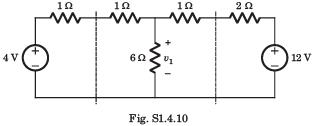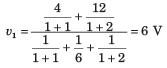Courses

# Test: Networks Theorem Part - 1

## 10 Questions MCQ Test Networking Theory | Test: Networks Theorem Part - 1

Description
This mock test of Test: Networks Theorem Part - 1 for Electrical Engineering (EE) helps you for every Electrical Engineering (EE) entrance exam. This contains 10 Multiple Choice Questions for Electrical Engineering (EE) Test: Networks Theorem Part - 1 (mcq) to study with solutions a complete question bank. The solved questions answers in this Test: Networks Theorem Part - 1 quiz give you a good mix of easy questions and tough questions. Electrical Engineering (EE) students definitely take this Test: Networks Theorem Part - 1 exercise for a better result in the exam. You can find other Test: Networks Theorem Part - 1 extra questions, long questions & short questions for Electrical Engineering (EE) on EduRev as well by searching above.
QUESTION: 1

### vTH, RTH, = ?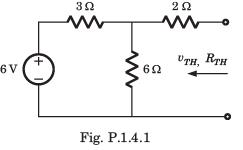Solution: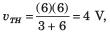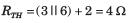QUESTION: 2

### iN, RN = ?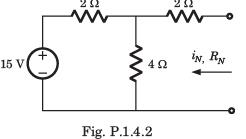Solution: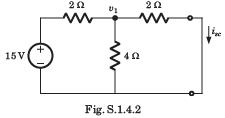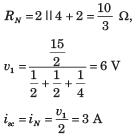QUESTION: 3

### vTH, RTH = ?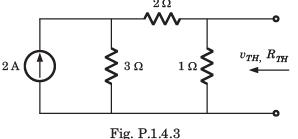Solution: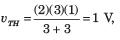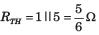QUESTION: 4

A simple equivalent circuit of the 2 terminal network shown in fig. P1.4.4 is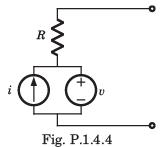Solution:

After killing all source equivalent resistance is R Open circuit voltage = v1

QUESTION: 5

iN, RN, = ?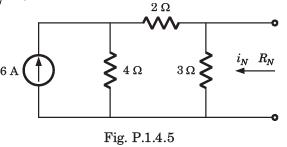Solution:

The short circuit current across the terminal is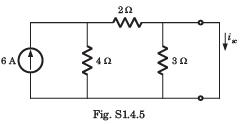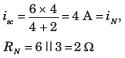QUESTION: 6

vTH, RTH = ?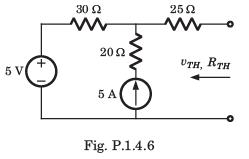Solution:

For the calculation of RTH if we kill the sources then 20 Ω resistance is inactive because 5 A source will be open circuit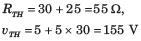QUESTION: 7

RTH = ?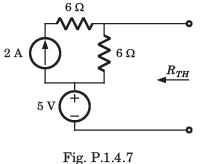Solution:

After killing the source, RTH = 6 Ω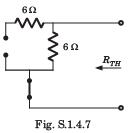QUESTION: 8

The Thevenin impedance across the terminals ab of the network shown in fig. P.1.4.8 is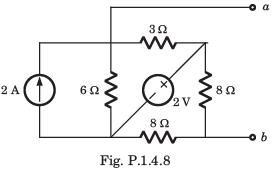Solution:

After killing all source,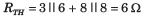QUESTION: 9

For In the the circuit shown in fig. P.1.4.9 a network and its Thevenin and Norton equivalent are given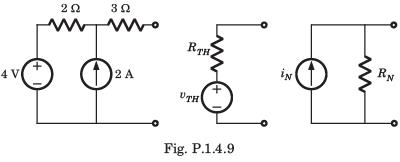The value of the parameter are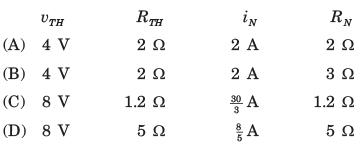Solution: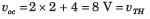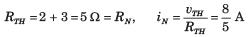QUESTION: 10

v1 = ?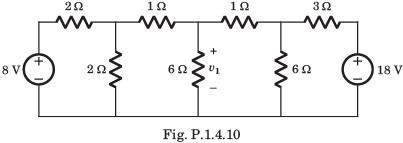Solution:

If we solve this circuit direct, we have to deal with three variable. But by simple manipulation variable can be reduced to one. By changing the LHS and RHS in Thevenin equivalent